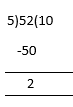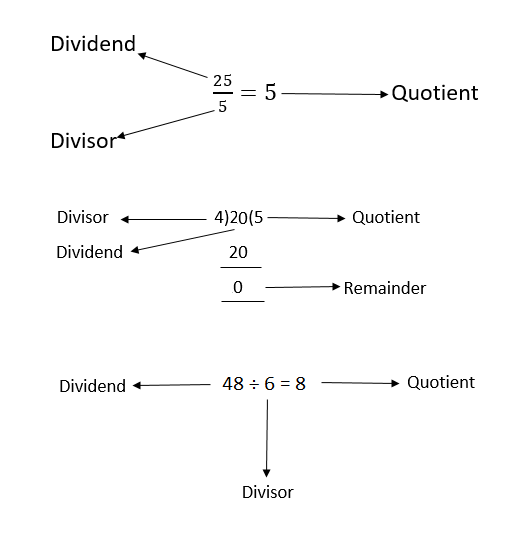# Divisor

In Mathematics, Divisor means a number which divides another number. It is a part of the division process. In division, there are four significant terms which are commonly used, such as Dividend, Divisor, Quotient and Remainder. You will learn the definitions of these terms in this article.

In Mathematics, we perform the most basic operations, which are addition, subtraction, division and multiplication. These arithmetic operations have been taught in our primary classes. Here, you will learn about the division factor, known as the divisor.
Dividend ÷ Divisor = Quotient

## Divisor Meaning

In division, we divide a number by any other number to get another number as a result. So, the number which is getting divided here is called the dividend. The number which divides a given number is the divisor. And the number which we get as a result is known as the quotient. The divisor which does not divide a number completely produces a number, which is referred to as remainder.

## Divisor Formula

The operation of division in the form of:
Dividend ÷ Divisor = Quotient

The above expression can also be written as:

Divisor = Dividend ÷ Quotient

Here, ‘÷’ is the symbol of division. But sometimes, it is also represented by the ‘/’ symbol, such as

Dividend / Divisor = Quotient

### Examples

1. In 22 ÷ 2 = 11, 22 is the dividend, 2 is the divisor and 11 is the quotient.
2. If, 45/5 = 9, then 5 is the divisor of 45, which divides number 45 into 9 equal parts.
3. 1 ÷ 2 = 0.5, the divisor 2 divides the number 1 into fraction.
4. In the below-given example, 5 is the divisor, 52 is the dividend, 10 is the quotient and 2 is the remainder.### How to represent the Divisor?

We can represent the divisor in three different ways. These are given in the figure below:### Key Points to Remember

• Divisor divides the number into parts.
• The divisor can divide the dividend either completely or partially. When divided completely, the remainder is zero and when divided partially, the remainder is a non-zero integer.
• Divisor could be a positive or negative number.
• A number that divides an integer exactly, leaving no remainder, is also termed as the divisor.
• The divisor 1 and -1 can divide every integer, present in the number line.

### Divisors of 18

We know that the numbers which divide the given number exactly are referred to as factors. These are also called divisors. From this, we can write the divisors of 18 as given below:

Factors of 18:

1 × 18 = 18

2 × 9 = 18

3 × 6 = 18

Factors are 1, 2, 3, 6, 9  and 18.

Therefore, the divisors of 18 are 1, 2, 3, 6, 9, and 18.

### Divisors of 26

The divisors of 26 are the numbers which divide it exactly without leaving any remainder.

Factors of 26:

1 × 26 = 26

2 × 13 = 26

Hence, the divisors of 26 are 1, 2, 13 and 26.

## Frequently Asked Questions on Divisor

Q1

### What is a divisor?

A divisor is an integer that divides another integer to produce a result. The number which is divided is called the dividend and the result obtained here is the quotient.
Dividend ÷ Divisor = Quotient

Q2

### Is a number a divisor of itself?

Yes, a number is a divisor of itself, which results in quotient 1. For example, 4 is divided by 2 and 4 itself.
4 ÷ 4 = 1

Q3

### Can a divisor be negative?

Divisors can be both positive and negative, but usually, we consider positive terms for divisors. For example, 4 is divisible by six divisors: -2, -1, -4, and 2, 1, 4. But only positive integers are considered here.

Q4

### What is the divisor in a fraction?

A fraction is represented in the form of p/q. So, p, which is the numerator here, is considered as dividend and q, which is the denominator here, is considered as the divisor.

Q5

### Is 1 a prime divisor?

No, it is not. To be a prime divisor a number has to meet the prime number condition, according to which there should be a minimum of two factors.